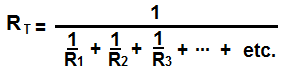﻿ Parallel and Series Resistor Calculator ﻿# Parallel and Series Resistor Calculator

### Parallel Resistor CalculatorR1: Ω (ohms) KΩ (Kilohms) MΩ (Megohms) R2 Ω (ohms) KΩ (Kilohms) MΩ (Megohms) R3 Ω (ohms) KΩ (Kilohms) MΩ (Megohms) R4 Ω (ohms) KΩ (Kilohms) MΩ (Megohms) R5 Ω (ohms) KΩ (Kilohms) MΩ (Megohms) R6 Ω (ohms) KΩ (Kilohms) MΩ (Megohms) R7 Ω (ohms) KΩ (Kilohms) MΩ (Megohms) R8 Ω (ohms) KΩ (Kilohms) MΩ (Megohms) R9 Ω (ohms) KΩ (Kilohms) MΩ (Megohms) R10 Ω (ohms) KΩ (Kilohms) MΩ (Megohms)

This parallel resistor calculator calculates the total parallel resistance of a circuit. This calculator allows up to 10 different resistor values. If you want to compute the total resistance of less than 10 resistors, just insert the values of the resistors you have and leave the rest of tbe fields blank. For example, if you want to compute the parallel resistance of 3 resistors, just insert values into the first 3 boxes and leave the rest blank. The calculator will give you the total parallel resistance for those 3 resistors. If you want to compute the parallel resistance of more than 10 resistors, then just start with the first 10 resistors and then calculate the equivalent parallel resistance. Once you have this result, place it into one of the boxes and insert into the other boxes the remaining resistors you have in parallel. The result will be the same as if you calculated all the resistors at once.

For this calculator, the user has the option of choosing whether the resistance is in ohms(Ω), kilohms (KΩ), or megohms (MΩ). 1 kilohm equals 1000Ω. 1 megohm equals 1,000,000Ω. The user can enter any resistance value in any unit value.

This parallel resistor calculator calculates the total resistance, based on the formula above. The unit of the result which it gives is in unit ohms (Ω).

### Series Resistor CalculatorR1: Ω (ohms) KΩ (Kilohms) MΩ (Megohms) R2 Ω (ohms) KΩ (Kilohms) MΩ (Megohms) R3 Ω (ohms) KΩ (Kilohms) MΩ (Megohms) R4 Ω (ohms) KΩ (Kilohms) MΩ (Megohms) R5 Ω (ohms) KΩ (Kilohms) MΩ (Megohms) R6 Ω (ohms) KΩ (Kilohms) MΩ (Megohms) R7 Ω (ohms) KΩ (Kilohms) MΩ (Megohms) R8 Ω (ohms) KΩ (Kilohms) MΩ (Megohms) R9 Ω (ohms) KΩ (Kilohms) MΩ (Megohms) R10 Ω (ohms) KΩ (Kilohms) MΩ (Megohms)

This series resistor calculator calculates the total series resistance of a circuit. This calculator allows up to 10 different resistor values. If you want to compute the total resistance of less than 10 resistors, just insert values of the resistors you have and leave the rest of the fields blank. For example, if you want to compute the series resistance of 3 resistors, just insert values into the first 3 boxes and leave the rest blank. The calculator will give you the total series resistance for those 3 resistors. If you want to compute the series resistance of more than 10 resistors, then just start with the first 10 resistors and then calculate the equivalent series resistance. Once you have this result, place it into one of the boxes and insert into the other boxes the remaining resistors you have in series. The result will be the same as if you calculated all the resistors at once.

Just like the other calculator, the user has the option of choosing whether the resistance is in ohms(Ω), kilohms (KΩ), or megohms (MΩ). The user can enter any resistance value in any unit value, whether ohms, kilohms, or megohms.

This series resistor calculator calculates the total resistance, based on the formula above. The unit of the result which it gives is unit ohms (Ω).

Related Resources

﻿